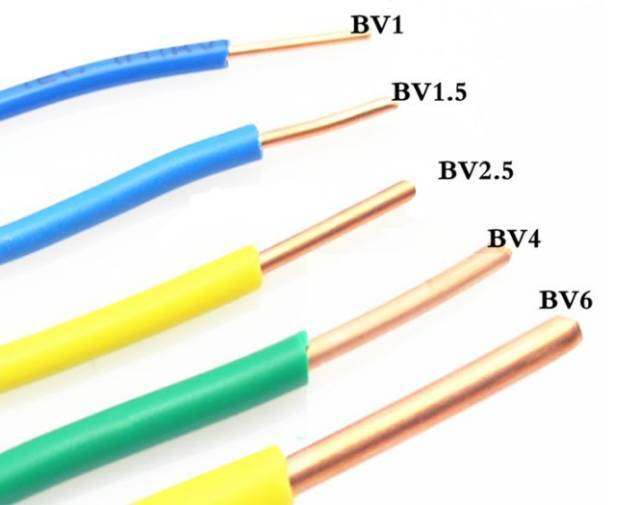E-mail us for a Quote:
Call us now:
+86-755-83261869

# Technical Article

## What is the diameter of a wire? How is this determined?

Time：2018-12-06 19:20:22 Click：

If you know the square of wire, you can calculate the radius of wire by using that the formula for calculating the circular area, then diameter = 2 * radius ;

Likewise, if you know the diameter of the wire, you can also calculate the square of wire by using that the same formula for calculating the circular area.

The Formula:

The Square of Wire=3.14* the Square of RadiusRelated articles：

Questions

E-MAIL